MainTutorialsOrganic ChemistryPractice TestsOnline QuizzesReference ToolsU.S. National Chemistry Olympiad: 1987 National Test

1. Assume, as Dalton did, that the atomic weight of oxygen is 7. A sample of 11 g of a uranium oxide contains 10 grams of U. Which of the following is compatible with this data?

(A) Uranium oxide is UO and the atomic weight of U is 70.
(B) Uranium oxide is U3O8, and the atomic weight of U is 70.
(C) Uranium oxide is UO2 and the atomic weight of U is 240.
(D) Uranium oxide is U2O3 and the atomic weight of U is 240.

2. The mass of a 6-C-12 atom is 19.92679 x 10�24 g and that of a 2-He-4 atom is 6.64658 x 10�24 g. If the international atomic weight scale had defined the mass of a 2-He-4 atom as exactly 4.0, what would be the atomic weight of Li which is 6.941 on the present scale?

(A) 6.361
(C) 6.936
(B) 6.941
(D) 6.946

3. Separation of the isotopes of uranium requires a physical method rather than a chemical method because

(A) it is too dangerous to mix other chemicals with uranium.
(B) the isotopes are chemically the same element.
(C) the isotopes differ in number of neutrons.
(D) natural uranium contains only 0.7% U-235.

4. The symbol of the element whose nucleus contains 27 neutrons and 22 protons is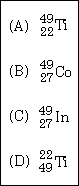5. Analysis of a quantity of it compound shows that it contains 0.110 mol of C, 0.055 mol of N, and 0.165 mol of 0. Its molecular weight is about 270. How many atoms of carbon are there in the empirical formula for the compound and how many in the molecular formula?

(A) Empirical - 1. molecular - 3
(B) Empirical - 2, molecular - 2
(C) Empirical - 2. molecular - 6
(D) Empirical - 3, molecular - 2

6. A phenomenon that suggests that light is emitted in packets called quanta is

(A) Hess's Law.
(B) diffraction of light.
(D) electron diffraction.

7. The observation that electrons can be diffracted is evidence that electrons

(A) have particulate properties.
(B) have wave properties.
(C) are emitted by atoms.
(D) are absorbed by.ions.

8. An electron can move from one orbital of a hydrogen atom to another. In which movement will the photon of highest energy be emitted?

(A) 2p ---> 1s
(B) 1s ---> 2p
(C) 3s ---> 2s
(D) 2s ---> 3s

9. The energy in joules of a photon of radiation of wavelength 1.23 x 10�5 m is

(A) (6.63 x 10�34)(3.00 x 108) / (1.23 x 10�5)
(B) (6.63 x 10�34)(1.23 x 10�5)
(C) (3.00 x 108) / (1.23 x 10�5)
(D) (1.23 x 10�5) / (6.63 x 10�34)

10. Which has the greatest ionization energy?

(A) He
(B) Ne
(C) Ar
(D) Cl�

11. Which is the smallest?

(A) K
(B) K+
(C) Ca
(D) Ca2+

12. Which bond would be least polar?

(A) H-F
(B) B-F
(C) Cl-F
(D) Ca-F

13. Which end of the bonds Si-Cl. At-Br, and Hg-P are positively charged?

(A) Si, At, P
(B) Cl, At, Hg
(C) Si, At, Hg
(D) Cl, Br, P

14. Assuming the VSEPR theory, which of the following molecules or ions would you expect to be polar?

(A) GeCl22
(B) GaF2+
(C) Pb(CH3)4
(D) GeF4

15. The neutral molecule XCl3 is found to have zero dipole moment. The element X is

(A) B
(B) N
(C) P
(D) I

16. The approximate OSO angle in the SO2 molecule is

(A) 90�
(B) 109�
(C) 120�
(D) 180�

17. The geometry of a molecule is best described in terms of the locations of the atomic nuclei. What geometries are possible for compunds whose bonding can be described using sp3 orbitals?

(A) Trigonal planar or bent
(B) Tetrahedral, bent, or trigonal bipyramidal
(C) Square planar, tetrahedral, or trigonal planar
(D) Trigonal bipyramidal or seesaw

18. A solution of which compund in water will best conduct electricity?

(A) CH3OCH3
(B) H2SO4
(C) NH3
(D) C6H6 (benzene)

19. Which contains the shortest OO bond?

(A) O2
(B) O3
(C) O22
(D) HO2

20. What is the probable shape of RnCl3+?

(A) Planar triangular
(B) T-shaped
(C) Pyramidal
(D) Square pyramidal

21. The length of the S-S bond in S2O32� is 2.01 A, in S2O42� is 2.39 A, in S2 is 1.89 A, and in S8 is 2.07 A. Which compound will require the most energy to break the S-S bond?

(A) S2O32
(B) S2O42
(C) S2
(D) S8

22. Which species would be a free radical?

(A) N2O
(B) NO2
(C) NO2+
(D) NO

23. Which will be about equally soluble in water or in 0.1 M HCl?

(A) NaClO4
(B) MgCO3
(C) PbCl2
(D) Al2O3

24. The oxidation state of which element is normally 2+, but can be as large as 7+?

(A) Calcium
(B) Manganese
(C) Fluorine
(D) Tin

25. A colorless crystalline solid melts below 150 �C. and dissolves in water to give a nonconducting solution. The solid may be

(A) salt, NaCl
(B) sand, SiO2
(C) propane, C3H8
(D) glucose, C6H12O6

26. The products of the electrolysis of molten MgBr2 are

(A) H2(g) and O2(g)
(B) H2(g) and Br2(g)
(C) Mg(l) and O2(g)
(D) Mg(l) and Br2(g)

27. SO2 is an example of

(A) a non-polar gaseous substance
(B) a strong oxidizing agent
(C) an acid anhydride
(D) a salt

28. In the reaction between the hydrogen sulfate ion and water, HSO4� ---> H3O+ + SO42�, the water acts as

(A) an acid
(B) a base
(C) a salt
(D) an inert medium

29. An amphoteric substance

(A) has neither acid or base properties
(B) turns litmus paper red and blue
(C) is insoluble in base, but dissolves in an acid
(D) reacts with both an acid and a base

30. The strongest acid is

(A) HClO4
(B) HClO3
(C) HClO2
(D) HClO

31. Which substance, when added to water, will not change the pH?

(A) NaHCO3
(B) NH4Cl
(C) KCN
(D) KCl

32. If a weak monoprotic acid is 5.0% dissociated in a 0.10 M aqueous solution of the acid, what is the acid equilibrium constant, Ka, for this acid?

(A) 2.5 x 10�5
(B) 2.5 x 10�4
(C) 5.0 x 10�3
(D) 5.0 x 10�2

33. Benzoic acid has a Ka = 6.6 x 10�5 . What is the pH of a 0.30 M aqueous solution of benzoic acid?

(A) 0.52
(B) 2.4
(C) 4.2
(D) 4.7

34. Forty milliliters (40.00 mL) of 0.0900 M NaOH is diluted to 100 mL and 30.00 mL of 0.1000 M HCl are added. The pH of the resulting solution is

(A) 11.66
(B) 12.38
(C) 12.18
(D) 9.57

35. Carbonic acid (aqueous carbon dioxide) is a diprotic acid which dissociates according to the steps

 H2CO3 + H2O <===> HCO3� + H3O+ Ka1 = 4.2 x 10�7 HCO3� + H2O <===> CO32� + H3O+ Ka2 = 4.8 x 10�11

What is the concentration of carbonate ion in a saturated solution of H2CO3 that is 0.037 M?

(A) 5.2 x 10�19 M
(B) 4.8 x 10�11 M
(C) 7.6 x 10�8 M
(D) 4.2 x 10�7 M

36. The true percentage of Na2CO3 in a sample of soda ash is 40.24%. A 0.4134 g sample was analyzed by titration with HCl. The sample required 28.56 ml of 0.1106 M HCl for complete neutralization. The equivalent weight of Na2CO3 is 53.00. The relative error, in ppt (parts per thousand) would be

(A) 13 ppt
(B) 6.5 ppt
(C) 6.4 ppt
(D) 12.8 ppt

37. The advice is often given that the bottom of a meniscus should be used in reading a volumetric instrument. In which of the following operations would it make no difference what part of the meniscus was used?

(A) Making a solution of known concentration using a volumetric flask.
(B) Transferring 5.00 ml of a solution using a transfer pipet.
(C) Transferring 23.7 of a solution using a burret.
(D) Measuring 65 ml using a measuring cylinder

38. When ammonia is added to a solution, a brown precipitate forms and the suernatant liquor turns deep blue. Which of the following best explains these phenomena?

(A) The solution contains Cu2+ and Ni2+
(B) The solution contains Fe3+ and Cu2+
(C) The solution contains Ni2+ and Fe3+
(D) The solution contains Fe2+ and Fe3+

39. Which salt is the least soluble?

(A) AgF
(B) AgCl
(C) AgBr
(D) AgI

40. Which salt is least likely to be dissolved in water if sulfuric acid is added to the water?

(A) CaCO3
(B) BaSO4
(C) Cu(OH)2
(D) NaCl

41. What is the pH of a saturated solution of milk of magnesia, Mg(OH)2 , if the Ksp of this compound is 1.2 x 10�11?

(A) 3.5
(B) 9.2
(C) 10.5
(D) 10.9

42. Inorganic cations often can be removed from solutionas insoluble sulfides by adding H2S which ionizes in water to form small concentrations of S2�(aq). What is the maximum concentration of Cu2+ that can exist in a solution containing 3.0 x 10�6 M sulfide ion (Ksp (CuS) = 8.5 x 10�45)?

(A) 2.6 x 10�50
(B) 2.8 x 10�39
(C) 5.3 x 10�20
(D) 8.2 x 10�14

43. A mixture of hydrogen and nitrogen are allowed to come to equilibrium at a specific temperature according to the reaction

3 H2 + N2 <===> 2 NH2

Analysis of the equilibrium mixture shows that it contains 1.5 mol NH3, 2.0 mol N2, and 3.0 mol H2. How many mols of H2 were present at the beginning of the reaction?

(A) 3.0
(B) 4.0
(C) 4.5
(D) 5.3

44. If the temperature is raised in an equilibrium system containing H2, N2 and NH3, it is found that the equilibrium constant for the formation of NH3 decreases. This indicates that the synthesis of ammonia from its elements

(A) is exothermic
(B) is endothermic
(C) is impractical
(D) occurs without any charges in energy.

45. Bleaching powder reacts with iodide ion according to the following unbalanced equation:

OCl� + I� + H+ <===> I2 + Cl� + H2O

A 0.6000 g sample of bleaching powder requires 35.24 mL of 0.1084 N Na2S2O3 to titrate the liberated iodine. The percentage of Cl in the sample is

(A) 22.58% Cl
(B) 5.15% Cl
(C) 11.29% Cl
(D) 45.16% Cl

46. The balanced oxidaton-reduction reaction for the following equation

Hg2+(aq) + H2S(aq) ---> Hg�(s) + S8(s) (acid solution)

would be

(A) 16 Hg2+(aq) + 16 H2S(aq) ---> 2 S8(s) + 16 Hg�(s) + 16 H+(aq)
(B) 8 Hg2+(aq) + 8 H2S(ag) ---> S8(s) + 16 Hg�(s) + 16 H+(aq)
(C) 8 Hg2+(aq) + 8 H2S(aq) ---> S8(s) + 8 Hg�(s) + 8 H+(aq)
(D) 8 Hg2+(aq) + 8 H2S(aq) ---> S8(s) + 8 Hg�(s) + 16 H+(aq)

[Reader's Note: Problem 46 in the original has Hg2+ in the problem statement, but Hg22+ in the answers. I have decided to go with the problem statement.]

47. The balanced equation for the reduction of the nitrate anion by the Fe(II) ion in an acidic solution is

(A) 3 Fe2+(aq) + NO3�(aq) + 4 H+(aq) ---> 3 Fe3+(aq) + NO(g) + 2 H2O(l)
(B) Fe2+(aq) + NO3�(aq) + 8 H+(aq) ---> Fe3+(aq) + NO(g) + 4 H2O(l)
(C) 2 Fe2+(aq) + 2 NO3�(aq) + 4 H+(aq) ---> 2 Fe3+(aq) + 2 NO(g) + 4 H2O(l)
(D) 3 Fe3+(aq) + NO(g) + 2 H2O(l) ---> 3 Fe3+(aq) + NO3�(aq) + 4 H+(aq)

48. Given the following half-reactions:

 A2+ + e� ---> A+ E� = +1.70 V B4+ + 2e� ---> B2+ E� = +0.60 V C3+ + e� ---> C2+ E� = +0.90 V

The only reaction that could be used in a titration would be

(A) A+ with B4+
(B) A+ with C3+
(C) A2+ with C2+
(D) C2+ with B4+

49. During a potentiometric oxidation-reduction titration, the only place at which the Ecell equals the E� of the substance being titrated is

(A) when the two half reactions are in equilibrium
(B) when twice the stoichiomeric amount of titrant has been added
(C) at the equivalence point
(D) half way to the equivalence point

50. Given the following standard half reaction Standard Electrode Potentials:

 Cd2+ + 2e� ---> Cd E� = -0.40 V Ag+ + e� ---> Ag E� = +0.80 V

The balanced spontaneous reaction involving silver and cadmium species would be

(A) 2 Ag�(s) + Cd�(aq) ---> 2 Ag+(aq) + Cd2+(aq)
(B) 2 Ag�(s) + Cd2+(aq) ---> 2 Ag+(ag) + Cd�(s)
(C) Cd�(s) + 2 Ag+(aq) ---> Cd2+(aq) + 2 Ag�(s)
(D) Cd�(s) + Ag+(aq) ---> Cd2+(aq) + Ag�(s)

51. Mercury batteries, like those used in electric watches, furnish a voltage of 1.35 V. If the overall oxidation-reduction equation taking place is

Zn�(s) + HgO(s) + 2H2O(l) ---> Zn(OH)2(s) + Hg�(l)

the anode reaction must be

(A) HgO(s) + 2H2O(l) + 2e� ---> Hg�(l) + 2 OH�(aq)
(B) Zn�(s) + 2 OH�(aq) ---> Zn(OH)2(s) + 2e�
(C) Hg�(l) + 2 OH�(aq) ---> HgO(s) + 2H20(l) + 2e�
(D) Zn(OH)2(s) + 2e� ---> Zn�(s) + 2 OH�(aq)

52. The following cell reaction furnishes 0.62V.

2Fe3+(aq) + Sn2+(aq) ---> 2 Fe2+(aq) + Sn4+(aq)

The maximum electrical energy per mole of Fe(III) ion is (1F = 9.65 x 104 C)

(A) 1.197 x 105 J/mole Fe(III) ion
(B) 2.394 x 105 J/mole Fe(III) ion
(C) 5.98 x 105 J/mole Fe(III) ion
(D) 5.98 x 104 J/mole Fe(III) ion

53. Aluminum oxide may be electrolyzed at 1000 �C to furnish aluninum metal. The cathode reaction is

Al3+ + 3e� ---> Al�

To prepare 5.12 kg of aluminum metal by this method would require

(A) 5.49 x 107 C of electricity
(B) 1.83 x 107 C of electricity
(C) 5.49 x 104 C of electricity
(D) 5.49 x 101 C of electricity

54. A sample of water vapor in the flask below is at a temperature of 65 �C and a pressure of 80 mmHg.If the flask is cooled to 45 �C, which of the following flasks best represents the sample under the new conditions? (Note: the vapor pressure of waterat 65 �C is 187 torr and at 45 �C is 72 torr.)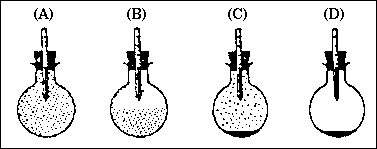55. Assuming ideal behavior, which of the following aqueous solutions will have the lowest vapor pressure at 25 �C?

(A) 0.800 molal C12H22O11
(B) 6.00 g of urea (NH2CONH2) dissolved in 100. g of H2O
(C) 5.00 g of NaCl dissolved in 100. g of H2O
(D) cC6H12O6 = 0.0200

56. A sample of water vapor in the flask below is at a temperature of 65 �C. A 7.00 gram sample of a compound was dissolved in 250 g of benzene . The observed freezing point of the solution was 4.20 �C. Determine the molecular weight of the compound. (Note: the normal freezing point of benzene is 5.5 �C and the kf = 5.12 �C / m)

(A) 27.6 g / mol
(B) 34.1 g / mol
(C) 110 g / mol
(D) 140 g / mol

57. Very careful measurement showed that a 0.1000 m solution of potassium sulfate has a freezing point of -0.4315 �C .What is the percent dissociation of potassium sulfate (K2SO4) in this solution?

(A) 23.20%
(B) 66.00%
(C) 77.33%
(D) 100% (effectively)

58. Consider the following initial arrangement in which the membrane is only permeable to water.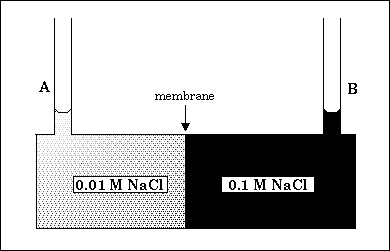What will be the final arrangement?

(A) Level B will be higher than level A, because water will flow from a region of high vapor pressure to a region of low vapor pressure.
(B) Level B will be higher than level A, because water will flow from a region of low vapor pressure to a region of high vapor pressure.
(C) Level A will be higher than level B, because water will flow from a region of low vapor pressure to a region of high vapor pressure.
(D) Level A will be higher than level B, because water will flow from a region of high vapor pressure to a region of low vapor pressure.

59. A 0.1375 g sample of solid magnesium is burned in a constant-volume bomb calorimeter that has a heat capacity of 1769 J / �C. The calorimeter contains exactly 1000 g of water and the temperature increases by 0.590 �C. The heat of combustion of Mg is (Note: the heat capacity of water is 4.184 J / g �C.)

(A) - 3.512 kJ.mol.
(B) - 25.5 kJ/mol
(C) - 289 kJ/mol
(D) -621 kJ/mol

60. We have a glass containing 200 grams of water at 25 �C and a supply of ice at 0 �C. The enthalpy of fusion for ice is DHfus = 6.01 kJ/mol and the specific heat of water is 4.184 J / g �C. How many grams of ice need be added (and melted) to the glass to reduce the temperature to 0 �C?

(A) 5.73 g
(B) 47.0 g
(C) 62.6 g
(D) 129 g

61. For the reaction in which ozone is formed in the upper atmosphere, O2(g) + O (g) ---> O3, DH= -105 kJ. DH�f for O3 = 143 kJ. The ionization energy of O3 is 1235 kJ/mol. DH�f for O(g) is

(A) 248 kJ
(B) 38 kJ
(C) 0 kJ
(D) - 248 kJ

62. Calculate the bond energy of the B-F bond in BF3 (boron trifluoride) gas.

DH�f of atomic B = 134.5 kcal/mol
DH�f of atomic F = 18.86 kcal/mol
DH�f of BF3 = - 271.75 kcal/mol

(A) 97.7 kcal/mol
(B) 116.6 kcal/mol
(C) 135.4 kcal/mol
(D) 154.3 kcal/mol

63. From the following data,

 C(graphite) + O2(g) ---> CO2(g) DH� = - 393.5 kJ H2(g) + 1/2 O2 (g) ---> H2O(l) DH� = -285.8 kJ 2 C2H6 + 7 O2(g) ---> 4 CO2(g) + 6 H2O(l) DH� = - 3120 kJ

the enthalpy of formation of C2H6(g) is

(A) -6409 kJ.
(B) -168 kJ.
(C) -84.4 kJ.
(D) 2441 kJ.

64. Which process will be accompained by a positive DG� at 25 �C?

(A) 2 Cl(g) ---> Cl2(g)
(B) HClO4(aq) + OCl�(aq) ---> ClO4�(aq) + HOCl(aq)
(C) 2 NaCl(l) ---> 2 Na(l) + Cl2(g)
(D) Cl2(aq) + 2 Br�(aq) ---> 2 Cl�(aq) + Br2(aq)

65 Given the following data

 Substance DH� (kJ/mol) S� (J/mol K) DG� (kJ/mol) FeO(s) - 266.3 57.49 - 245.12 C(graphite) 0 5.74 0 Fe(s) 0 27.28 0 CO(g) - 110.5 197.6 - 137.15

determine at what temperature the following reaction is spontaneous.

FeO(s) + C(graphite) -------> Fe(s) + CO (g)

(A) 298K
(B) 668K
(C) 966K
(D) Because DG� is positive, the reaction will never be spontaneous.

66. The decomposition of NO

2 NO(g) ------> N2(g) + O2(g)

follows simple second order kinetics. In a particular experiment, it takes 2000 seconds for the concentration of NO to fall from 2.80 x 10�3 M. If a second experiment is perfomed with the initial concentration of NO at 0.0820 M, calculate how long it will take for the concentration to fall to 4.10 x 10�2 M.

(A) 1.71 x 102 sec
(B) 1.00 x 103 sec
(C) 2.86 x 103 sec
(D) 4.12 x 103 sec

67. A proposed mechanism for the reaction

2 NO(g) + Br2(g) ---> 2 NOBr(g)

is as follows:

 NO(g) + Br2(g) ----> NOBr2(g) slow NO(g) + NOBr2(g) ----> 2NOBr(g) fast

The rate law is:

(A) rate = k[NO][Br2]
(B) rate = k[NO]2[Br2]
(C) rate = k[NO][NOBr2]
(D) rate = k[NO]2

68. At 100 �C, nitrogen dioxide decomposes according to the following equation

NO2(g) -------> NO(g) + 1/2 O2(g)

The reaction is studied by following the change of the NO2 concentration with time. The following three graphs were obtained.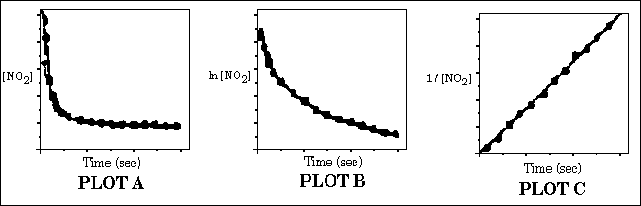Which of the following statements is false?

(A) The reaction follows first order kinetics.
(B) The reaction follows second order kinetics.
(C) The rate constant can be determined from the slope of the line in PLOT C.
(D) The initial rate for the reaction can be determined from PLOT A.

69. The rate of an elementary reaction

A(g) + B(g) -------> C(g) + D(g)

has been studied as a function of temperature between 5000 and 18000 K. The following data were obtained for the rate constant:

 T (K) k (M/sec) 5000 5.49 x 106 10000 9.86 x 108 15000 5.57 x 109 18000 9.92 x 109

Calculate the activation energy for the reaction.

(A) 25.9 kJ/mol
(B) 52.0 kJ/mol
(C) 359 kJ/mol
(D) 432 kJ/mol

70. Proteins are a class of poly molecules called polypeptides, a simple example of which is represented by the structural formula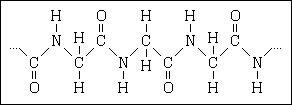The substances that serve as repeating units (monomers) in these polymers are classified as

(A) mineral acids
(B) carboxylicacids
(C) amino acids
(D) Nucleic acidsnews | about us | contact us tutorials index | organic chemistry | practice tests | online quizzes | reference tools site copyright (c) 2002-2013 Learn ChemSubscribe to our low volume newsletter to receive up-to-date information about the CHEM SITE